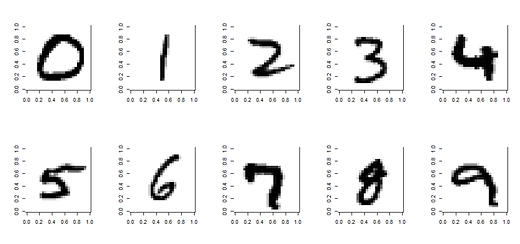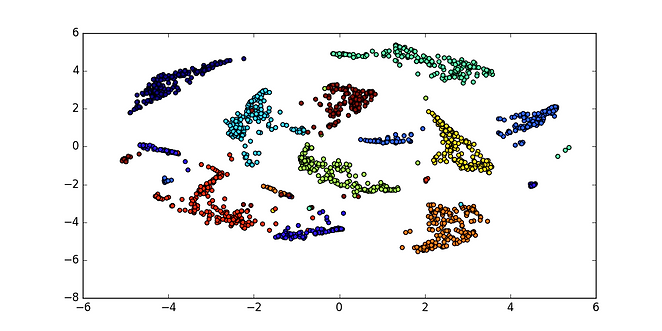# t-Distributed Stochastic Neighbor Embedding (t-SNE) in python

Codes related to present tutorial are available at GitHub Repository.

t-SNE is a tool for data visualization. It reduces the dimension of data to 2 or 3 dimensions so that it can be plotted easily. Local similarities are preserved by this embedding.

Human cannot visualize data more than 3-4 dimension easily. so by somehow we need to reduce such data into two or three dimensional data.

For t-SNE implementation in language of your choice, you  may visit Laurens van der Matten’s site.

For Python users, there is a PyPI package called tsne. You can install it easily with pip install tsne.

We will see use of TSNE with two different examples.

1. Iris Data-set

The Iris Data-set. This data sets consists of 3 different types of iris flower petals (Setosa, Versicolour, and Virginica) need to be separated on the basis of four features:

1. sepal length in cm
2. sepal width in cm
3. petal length in cm
4. petal width in cm

So this is four dimensional data and our task is to visualize all classes as clusters in two dimensional image. Following code will use T-SNE technique to visualize all 3 classes separately.

 import csv
import numpy as np
from matplotlib import pyplot as plt
from sklearn.manifold import TSNE

"""
:param filename:
:param numattrs: number of column in file, Excluding  class column
:return:
"""
csvfile = open(filename, 'r')
dataset = list(lines)
for x in range(len(dataset)):
for y in range(numattrs):
dataset[x][y] = float(dataset[x][y])
return dataset

X = np.asarray(XY)[:, :4]  # skipping class column
Y = np.asarray(XY)[:, 4:]  # taking only class column
# converting to numerical values
Y = reduce(lambda x, y: x + y, Y.tolist())  # flattening class values [[X],[Y],[X]] == > [X,Y,X]
Uniquelabels = list(set(Y))
# Finding Number of unique labels  [X,Y] will be having something this Set('Iris-setosa','Iris-versicolor','Iris-virginica')
# converting categorical class value to numerical one
YNumeric = []
for each in Y:
"""
This loop will convert categorical classes ('Iris-setosa','Iris-versicolor','Iris-virginica') to numerical one e.g. 1,2,3 respectively
"""
YNumeric.append(Uniquelabels.index(each))
# print YNumeric
# plotting after applying t-nse
X_tsne = TSNE(learning_rate=100).fit_transform(X)
plt.<p align="center">figure(figsize=(10, 5))</p>
plt.scatter(X_tsne[:, 0], X_tsne[:, 1], c=YNumeric)
plt.show()


I have plotted the 2D graph obtained after running above code and  it clearly shows 3 classes very distinctly separated from each other. For to cross verify I have kept only 5 samples of  Iris-virginica. Five Iris-virginica samples are separated correctly with violet colour in the below shown figure.Figure 1, Applying T-SNE to iris dataset

2. MNIST Data-setFigure 2. MNIST data-set representation

MNIST Digit data-set is already included in the sklearn package.  In MNIST data-set each digit is given in form of image of 8*8 pixels as shown in figure 2. MNIST data-set is in the form of a dictionary with two parts:

1. digit[‘images’],  1797 images of size 8*8  pixel represented by floats

2. digit[‘target’], image labels [1,2,3,4] represents digit present in given image.

TSNE don’t take 2-D arrays of 8*8 what we have right now in raw data-set, to make it compatible we will first flatten arrays to 1-D with 64 element into it. In code snippet line 10-15 will convert all 2-D data to 1-D and then we can have 64 dimensional data which may belongs to any of the 10 classes [1,2,3,…,9].

 from matplotlib import pyplot as plt
from sklearn import datasets
from sklearn.manifold import TSNE
# optional print statements
# print digits['images'], digits['target']
# print digits['images'].shape
# flattening the 2D Array to 1D Array
flatten = []
for eachDigit in digits['images']:
temp = []
for eachrow in eachDigit:
temp.extend(eachrow)
flatten.append(temp)
# plotting with t-nse
X_tsne = TSNE(learning_rate=100).fit_transform(flatten)
plt.figure(figsize=(10, 5))
plt.scatter(X_tsne[:, 0], X_tsne[:, 1], c=digits['target'])
plt.show()


We get following representation at the end. which clearly shows 10 different clusters, each representing single digit.Figure 2. MNIST data-set processed with TSNE

We will be  using the same visualization technique in upcoming tutorial of SMOTE.

Share: